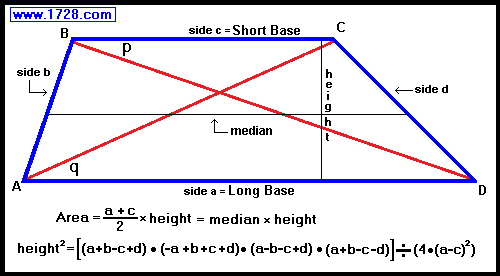# What Do You Know About Trapezoid?

Approved & Edited by ProProfs Editorial Team
At ProProfs Quizzes, our dedicated in-house team of experts takes pride in their work. With a sharp eye for detail, they meticulously review each quiz. This ensures that every quiz, taken by over 100 million users, meets our standards of accuracy, clarity, and engagement.
| Written by Tunde1995
T
Tunde1995
Community Contributor
Quizzes Created: 212 | Total Attempts: 5,663,011
Questions: 10 | Attempts: 168SettingsA trapezoid is shape with four sides. In mathematics, the knowledge of trapezoid is governed by two schools of thought viz: inclusive and exclusive definition. The former presents the trapezoid as a four-sided shape with only a pair parallel sides. The later presents it as a four-sided shape with more than one pair of parallel sides. Show your mastery of Euclidean geometry by answering the following questions.

• 1.

### How many triangles does the diagonals of trapezoid form?

• A.

Four triangles

• B.

Two triangles

• C.

A triangle

• D.

Six triangles

A. Four triangles
Explanation
The diagonals of a trapezoid form four triangles. The trapezoid has two bases and two legs. When the diagonals are drawn, they intersect at a point inside the trapezoid, creating four triangles. Each triangle is formed by connecting one of the endpoints of a base to the intersection point of the diagonals. Therefore, the correct answer is four triangles.

Rate this question:

• 2.

### What is the sum of the two cosines adjacent angle in a trapezoid?

• A.

1

• B.

2

• C.

90

• D.

0

D. 0
Explanation
In a trapezoid, the sum of the two adjacent angles is always equal to 180 degrees. Since the cosine of 180 degrees is equal to -1, the sum of the two cosines adjacent angles in a trapezoid is 0.

Rate this question:

• 3.

### ................... degree implies that the quadrilateral is a trapezoid:

• A.

180 degrees

• B.

170 degrees

• C.

165 degrees

• D.

45 degrees

A. 180 degrees
Explanation
A trapezoid is a quadrilateral with at least one pair of parallel sides. The sum of the interior angles of any quadrilateral is always 360 degrees. However, in a trapezoid, one pair of opposite angles are supplementary, meaning they add up to 180 degrees. Therefore, if one angle in a quadrilateral is 180 degrees, it implies that the quadrilateral is a trapezoid.

Rate this question:

• 4.

### A bimedian divides a trapezoid to how many parts?

• A.

Two parts

• B.

Four parts

• C.

Six parts

• D.

Eight parts

A. Two parts
Explanation
A bimedian divides a trapezoid into two parts. A bimedian is a line segment that connects the midpoints of the non-parallel sides of a trapezoid. It divides the trapezoid into two smaller trapezoids that are congruent to each other. Therefore, the correct answer is two parts.

Rate this question:

• 5.

### How many adjacent right angles does a right trapezoid has?

• A.

• B.

• C.

• D.

Explanation
A right trapezoid has two adjacent right angles. A right trapezoid is a quadrilateral with one pair of parallel sides and one pair of non-parallel sides. The non-parallel sides are typically referred to as the legs of the trapezoid, while the parallel sides are called the bases. Since a right trapezoid has one pair of parallel sides, it means that one pair of opposite angles are right angles. Therefore, there are two adjacent right angles in a right trapezoid.

Rate this question:

• 6.

### A trapezoid as a quadrilateral is _________?

• A.

Concave

• B.

Convex

• C.

Supplementary

• D.

Complementary

A. Concave
Explanation
A trapezoid is a convex quadrilateral, meaning that all of its interior angles are less than 180 degrees and all of its diagonals lie within the shape.

Rate this question:

• 7.

### The following are types of trapezoid except?

• A.

Right trapezoid

• B.

Acute trapezoid

• C.

Complementary trapezoid

• D.

Left trapezoid

C. Complementary trapezoid
Explanation
A trapezoid is a quadrilateral with at least one pair of parallel sides. A right trapezoid is a trapezoid where one of the angles is a right angle. An acute trapezoid is a trapezoid where all angles are acute angles. A left trapezoid is a trapezoid where the longer base is on the left side. However, there is no such thing as a complementary trapezoid. The term "complementary" refers to angles that add up to 90 degrees, which is not a characteristic of a trapezoid.

Rate this question:

• 8.

### A parallelogram is a trapezoid with _____ parallel sides?

• A.

Two

• B.

Eight

• C.

Four

• D.

Six

A. Two
Explanation
A parallelogram is a quadrilateral with both pairs of opposite sides parallel. A trapezoid, on the other hand, is a quadrilateral with only one pair of opposite sides parallel. Therefore, a parallelogram can also be considered a trapezoid with two parallel sides.

Rate this question:

• 9.

### Which of the following terms does not correlate with a trapezoid?

• A.

Incircle

• B.

Right angle

• C.

Vertice

• D.

Circumference

D. Circumference
Explanation
The term "circumference" does not correlate with a trapezoid. A trapezoid is a quadrilateral with one pair of parallel sides. The term "incircle" refers to a circle that is tangent to all four sides of a polygon, including a trapezoid. "Right angle" is a term that can be associated with a trapezoid if one of its angles measures 90 degrees. "Vertice" refers to the corners of a polygon, including a trapezoid. However, "circumference" is a term commonly used to describe the distance around a circle, which is not applicable to a trapezoid.

Rate this question:

• 10.

### The angles of a trapezoid obey which of these laws?

• A.

Pythagoras theorem

• B.

Sine law

• C.

Commutative law

• D.

Cosine lawBack to top